EnchantedLearning.com is a user-supported site.
As a bonus, site members have access to a banner-ad-free version of the site, with print-friendly pages.

 You might also like: Algebra Worksheets: Quadratic Equations with No Linear Term Algebra: Solve the quadratic equations Worksheet #6 Printout Algebra: Solve the quadratic equations Worksheet #1 Printout Multiply Polynomials: Algebra Algebra: Solve the quadratic equations Worksheet #3 Printout Today's featured page: Insect Math-Code Games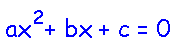More Math Activities EnchantedLearning.comAlgebra:Quadratic EquationsQuadratic Equation: ax2 - c = 0Printable Worksheets

A quadratic equation is an equation that has a second-degree term and no higher terms. A second-degree term is a variable raised to the second power, like x2. When you graph a quadratic equation, you get a parabola, and the solutions to the quadratic equation represent where the parabola crosses the x-axis.

A quadratic equation can be written in the form:,
where a, b, and c are numbers (a ≠0), and x is the variable. x is a solution (or a root) if it satisfies the equation ax2 + bx + c = 0.

Some examples of quadratic equations include:
 3x2 + 9x - 2 = 0 6x2 + 11x = 7 4x2 = 13

Some quadratic equations can be solved easily by factoring. Some simple-to-solve quadratic equations are:

 x2 - 1 = 0 (x + 1)(x - 1) = 0 x = ±1 x2 - 5x + 6 = 0 (x - 2)(x - 3) = 0 x = 2, 3

Most second-degree equations are more difficult to solve, and cannot be solved by simple factoring. The quadratic formula is a general way of solving any quadratic equation:This formula gives two solutions, although the two solutions may be the same number. (When solving any polynomial equation of degree n, there are at most n solutions to that equation.)

The quadratic formula is obtained by solving the general quadratic equation. This is one way to derive the quadratic formula: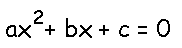Divide each side of the equation by a.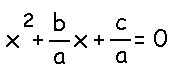Subtract c/a from each side of the equation.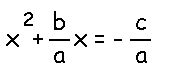Add (b/2a)2 to each side of the equation (to complete the square).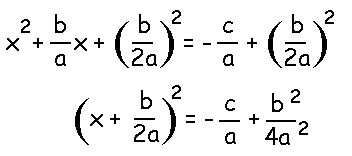Find a common denominator for the right side of the equation.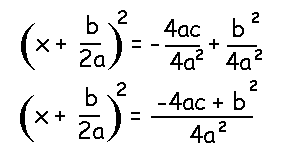Take the square root of each side of the equation.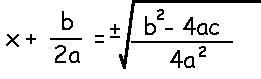Add b/2a to each side of the equation.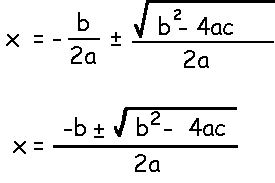The plus-or-minus sign shows that there are two possible solutions.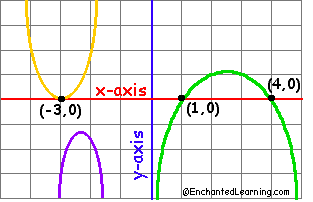The green parabola has 2 x-intercepts. Its corresponding quadratic equation has 2 distinct solutions (x=1 and x=4). The yellow parabola has 1 x-intercept. Its corresponding quadratic equation has 1 solution (x=-3). The purple parabola has no x-intercepts. Its corresponding quadratic equation has no solutions.
You can try to solve any quadratic equation by using the quadratic formula (or by solving it algebraically, similar to the way we just derived the quadratic formula above).

Every quadratic equation has at most two solutions, but for some equations, the two solutions are the same number, and for others, there is no solution on the number line (because it would involve the square root of a negative number).

One way to understand this visually is to realize that when you graph a quadratic equation (a second-degree equation), you get a parabola. When you set the quadratic equation equal to zero, this represents the points where the parabola hits the x-axis (the x-intercepts, where y=0).

Introduction to Algebra - Printable Worksheets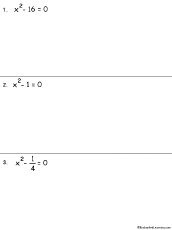Quadratic Equations Worksheets (with no linear term)Solve simple (pure) quadratic equations (no linear terms).

Enchanted Learning®
Over 35,000 Web Pages
Sample Pages for Prospective Subscribers, or click below

 Overview of Site What's New Enchanted Learning Home Monthly Activity Calendar Books to Print Site Index K-3 Crafts K-3 Themes Little ExplorersPicture dictionary PreK/K Activities Rebus Rhymes Stories Writing Cloze Activities Essay Topics Newspaper Writing Activities Parts of Speech Fiction The Test of Time iPhone app TapQuiz Maps - free iPhone Geography Game Biology Animal Printouts Biology Label Printouts Biomes Birds Butterflies Dinosaurs Food Chain Human Anatomy Mammals Plants Rainforests Sharks Whales Physical Sciences: K-12 Astronomy The Earth Geology Hurricanes Landforms Oceans Tsunami Volcano Languages Dutch French German Italian Japanese (Romaji) Portuguese Spanish Swedish Geography/History Explorers Flags Geography Inventors US History Other Topics Art and Artists Calendars College Finder Crafts Graphic Organizers Label Me! Printouts Math Music Word Wheels

## Enchanted Learning Search

 Search the Enchanted Learning website for: